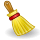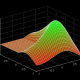# TUTORIAL MATLAB

MATLAB (matrix laboratory) is a numerical computing environment and fourth-generation programming language. Developed by MathWorks, MATLAB allows matrix manipulations, plotting of functions and data, implementation of algorithms, creation of user interfaces, and interfacing with programs written in other languages, including C, C++, Java, and Fortran.

Although MATLAB is intended primarily for numerical computing, an optional toolbox uses the MuPAD symbolic engine, allowing access to symbolic computing capabilities. An additional package, Simulink, adds graphical multi-domain simulation and Model-Based Design for dynamic and embedded systems.

In 2004, MATLAB had around one million users across industry and academia. MATLAB users come from various backgrounds of engineering, science, and economics. MATLAB is widely used in academic and research institutions as well as industrial enterprises.

## Cleve Moler, the chairman of the computer-science department at the University of New Mexico, started developing MATLAB in the late 1970s. He designed it to give his students access to LINPACK and EISPACK without their having to learn Fortran. It soon spread to other universities and found a strong audience within the applied mathematics community. Jack Little, an engineer, was exposed to it during a visit Moler made to Stanford University in 1983. Recognizing its commercial potential, he joined with Moler and Steve Bangert. They rewrote MATLAB in C and founded MathWorks in 1984 to continue its development. These rewritten libraries were known as JACKPAC. In 2000, MATLAB was rewritten to use a newer set of libraries for matrix manipulation, LAPACK.

MATLAB was first adopted by researchers and practitioners in control engineering, Little’s specialty, but quickly spread to many other domains. It is now also used in education, in particular the teaching of linear algebra and numerical analysis, and is popular amongst scientists involved in image processing.

## SyntaxThis article is written like a manual or guidebook. Please help rewrite this article from a neutral point of view. (October 2008)

The MATLAB application is built around the MATLAB language. The simplest way to execute MATLAB code is to type it in the Command Window, which is one of the elements of the MATLAB Desktop. When code is entered in the Command Window, MATLAB can be used as an interactive mathematical shell. Sequences of commands can be saved in a text file, typically using the MATLAB Editor, as a script or encapsulated into a function, extending the commands available.

### Variables

Variables are defined as the assignment operator, `=`. MATLAB is a weakly dynamically typed programming language. It is a weakly typed language because types are implicitly converted. It is a dynamically typed language because variables can be assigned without declaring their type, except if they are to be treated as symbolic objects, and that their type can change. Values can come from constants, from computation involving values of other variables, or from the output of a function. For example:

```>> x = 17
x =
17
>> x = 'hat'
x =
hat
>> y = x + 0
y =
104        97       116
>> x = [3*4, pi/2]
x =
12.0000    1.5708
>> y = 3*sin(x)
y =
-1.6097    3.0000```

### Vectors/matrices

As suggested by its name (a contraction of “Matrix Laboratory”), MATLAB can create and manipulate arrays of 1 (vectors), 2 (matrices), or more dimensions. In the MATLAB vernacular, a vector refers to a one dimensional (1×N or N×1) matrix, commonly referred to as an array in other programming languages. A matrix generally refers to a 2-dimensional array, i.e. an m×n array where m and n are greater than 1. Arrays with more than two dimensions are referred to as multidimensional arrays. Arrays are a fundamental type and many standard functions natively support array operations allowing work on arrays without explicit loops. Therefore the MATLAB language is also an example of array programming language.

A simple array is defined using the syntax: init`:`increment`:`terminator. For instance:

```>> array = 1:2:9
array =
1 3 5 7 9```

defines a variable named `array` (or assigns a new value to an existing variable with the name `array`) which is an array consisting of the values 1, 3, 5, 7, and 9. That is, the array starts at 1 (the init value), increments with each step from the previous value by 2 (the increment value), and stops once it reaches (or to avoid exceeding) 9 (the terminator value).

```>> array = 1:3:9
array =
1 4 7```

the increment value can actually be left out of this syntax (along with one of the colons), to use a default value of 1.

```>> ari = 1:5
ari =
1 2 3 4 5```

assigns to the variable named `ari` an array with the values 1, 2, 3, 4, and 5, since the default value of 1 is used as the incrementer.

Indexing is one-based, which is the usual convention for matrices in mathematics, although not for some programming languages.

Matrices can be defined by separating the elements of a row with blank space or comma and using a semicolon to terminate each row. The list of elements should be surrounded by square brackets: []. Parentheses: () are used to access elements and subarrays (they are also used to denote a function argument list).

```>> A = [16 3 2 13; 5 10 11 8; 9 6 7 12; 4 15 14 1]
A =
16  3  2 13
5 10 11  8
9  6  7 12
4 15 14  1

>> A(2,3)
ans =
11```

Sets of indices can be specified by expressions such as “2:4”, which evaluates to [2, 3, 4]. For example, a submatrix taken from rows 2 through 4 and columns 3 through 4 can be written as:

```>> A(2:4,3:4)
ans =
11 8
7 12
14 1```

A square identity matrix of size n can be generated using the function eye, and matrices of any size with zeros or ones can be generated with the functions zeros and ones, respectively.

```>> eye(3)
ans =
1 0 0
0 1 0
0 0 1
>> zeros(2,3)
ans =
0 0 0
0 0 0
>> ones(2,3)
ans =
1 1 1
1 1 1```

Most MATLAB functions can accept matrices and will apply themselves to each element. For example, `mod(2*J,n)` will multiply every element in “J” by 2, and then reduce each element modulo “n”. MATLAB does include standard “for” and “while” loops, but (as in other similar applications such as R), using the vectorized notation often produces code that is faster to execute. This code, excerpted from the function magic.m, creates a magic square M for odd values of n (MATLAB function meshgrid is used here to generate square matrices I and J containing 1:n).

```[J,I] = meshgrid(1:n);
A = mod(I+J-(n+3)/2,n);
B = mod(I+2*J-2,n);
M = n*A + B + 1;```

### Semicolons

Unlike many other languages, where the semicolon is used to terminate commands, in MATLAB the semicolon serves to suppress the output of the line that it concludes (it serves a similar purpose in Mathematica).

### Graphics

Function plot can be used to produce a graph from two vectors x and y. The code:

```x = 0:pi/100:2*pi;
y = sin(x);
plot(x,y)```

produces the following figure of the sine function:Three-dimensional graphics can be produced using the functions surf, plot3 or mesh.

 ```[X,Y] = meshgrid(-10:0.25:10,-10:0.25:10); f = sinc(sqrt((X/pi).^2+(Y/pi).^2)); mesh(X,Y,f); axis([-10 10 -10 10 -0.3 1]) xlabel('{\bfx}') ylabel('{\bfy}') zlabel('{\bfsinc} ({\bfR})') hidden off``` ```[X,Y] = meshgrid(-10:0.25:10,-10:0.25:10); f = sinc(sqrt((X/pi).^2+(Y/pi).^2)); surf(X,Y,f); axis([-10 10 -10 10 -0.3 1]) xlabel('{\bfx}') ylabel('{\bfy}') zlabel('{\bfsinc} ({\bfR})')``` This code produces a wireframe 3D plot of the two-dimensional unnormalized sinc function: This code produces a surface 3D plot of the two-dimensional unnormalized sinc function:### Structures

MATLAB supports structure data types. Since all variables in MATLAB are arrays, a more adequate name is “structure array”, where each element of the array has the same field names. In addition, MATLAB supports dynamic field names (field look-ups by name, field manipulations etc). Unfortunately, MATLAB JIT does not support MATLAB structures, therefore just a simple bundling of various variables into a structure will come at a cost.[citation needed]

### Function handles

MATLAB supports elements of lambda-calculus by introducing function handles, or function references, which are implemented either in .m files or anonymous/nested functions.

### Secondary programming

MATLAB also carries secondary programming which incorporates the MATLAB standard code into a more user friendly way to represent a function or system.

### Classes

Although MATLAB supports classes, the syntax and calling conventions are significantly different from other languages. MATLAB supports value classes and reference classes, depending if the class has handle as super-class (for reference classes) or not (for value classes).

Depending if a class is declared as value or reference, method call behavior is different. For example, a call to a method

`object.method();`

can alter any variables of object variable only for reference classes.

## Object-oriented programming

MATLAB’s support for object-oriented programming includes classes, inheritance, virtual dispatch, packages, pass-by-value semantics, and pass-by-reference semantics.

```classdef hello
methods
function doit(this)
disp('Hello!')
end
end
end```

When put into a file named hello.m, this can be executed with the following commands:

```>> x = hello;
>> x.doit;
Hello!

RELATED POST ::
KUIS PENJUMLAHAN (LATIHAN)
LATIHAN 3
Latihan Office mix
CONTOH ABSTRAK 2
CONTOH ABSTRAK 1
```Welcome to my blog. My name is Nico. Admin of this blog. I am a student majoring in mathematics who dreams of becoming a professor of mathematics. I live in Kwadungan, Ngawi, East Java. Hopefully in all the posts I can make a good learning material to the intellectual life of the nation. After the read, leave a comment. I always accept criticism suggestion to build a better me again .. Thanks for visiting .. : mrgreen:

Posted on August 13, 2011, in DUNIA MATEMATIKA and tagged , , , , , , , . Bookmark the permalink. 3 Comments.

1.matlabideas
2.MENONE